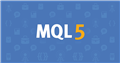# PrintFormat and StringFormat with variable precision

Hello folks,

According to C and C++ definitions, is possible to use printf with variables "width" and "precision".

In MQL documentation is clear that we can use variable "width", but is not clear to "precision". Is possible to use variable "precision"? Is there any way to do this?

https://www.mql5.com/en/docs/common/printformat

I'm using this code to test. Also, the variable "width" doesn't have the same behavior as the static one, see "strValue1" and "strValue3, is not possible to put the zeroes.

```   string strValue1 = StringFormat("Format test: %07.3f", 1.25);        // Result --> Format test: 001.250
string strValue2 = StringFormat("Format test: %*.3f", 7, 1.25);      // Result --> Format test:   1.250
string strValue3 = StringFormat("Format test: %*.3f", 07, 1.25);     // Result --> Format test:   1.250
string strValue4 = StringFormat("Format test: %*.*f", 07, 3, 1.25);  // Result --> Format test:  *.*f
```Documentation on MQL5: Common Functions / PrintFormat
• www.mql5.com
The number, order and type of parameters must exactly match the set of qualifiers, otherwise the print result is undefined. Instead of PrintFormat() you can use If the format string is followed by parameters, this string must contain format specifications that denote output format of these parameters. Specification of format always starts with...

you can do it this way (it's even shown in the help, F1):

```double val = 1.7612345679; // price
string fmt = StringFormat( "%%0%d.%df", 3+_Digits, _Digits ), // => "%08.5"
var = StringFormat(fmt,val);
=> 01.7612```

You can put it even into a function:

`string sPrintf(double val, int zeros, int digs) { string _=StringFormat("%%0%d.%df", zeros+1+digs, digs); return(StringFormat(_,val)); }`

(untested!)

Carl Schreiber:

you can do it this way (it's even shown in the help, F1):

You can put it even into a function:

(untested!)

Thanks @Carl Schreiber, your code works well, but it is not very practical if we use a more long expression. So, I tried compress all the code and I got:

```{
double A = 1.25;
double B = 3.45;
double C = 5.01;
int    z = 7;
int    d = 3;

// In MQL5 and Standard C (static precision):
string strValue5 = StringFormat("A: %7.3f, B: %7.3f, C: %7.3f", A, B, C);  // Result --> A:   1.250, B:   3.450, C:   5.010

// In Standard C (variable precision):
string strValue6 = StringFormat("A: %*.*f, B: %*.*f, C: %*.*f", z, d, A, z, d, B, z, d, C);  // Result --> A:  *.*f, B:  *.*f, C:  *.*f

// In MQL5 (variable precision):
string strValue7 = StringFormat("A: %s, B: %s, C: %s", prt(A, z, d) , prt(B, z, d), prt(C, z, d));  // Result --> A: 0000001.250, B: 0000003.450, C: 0000005.010
}

...

string prt(double val, int zeros, int digs)
{
string _=StringFormat("%%0%d.%df", zeros+1+digs, digs);
return(StringFormat(_,val));
}
```

Working very well, thanks!